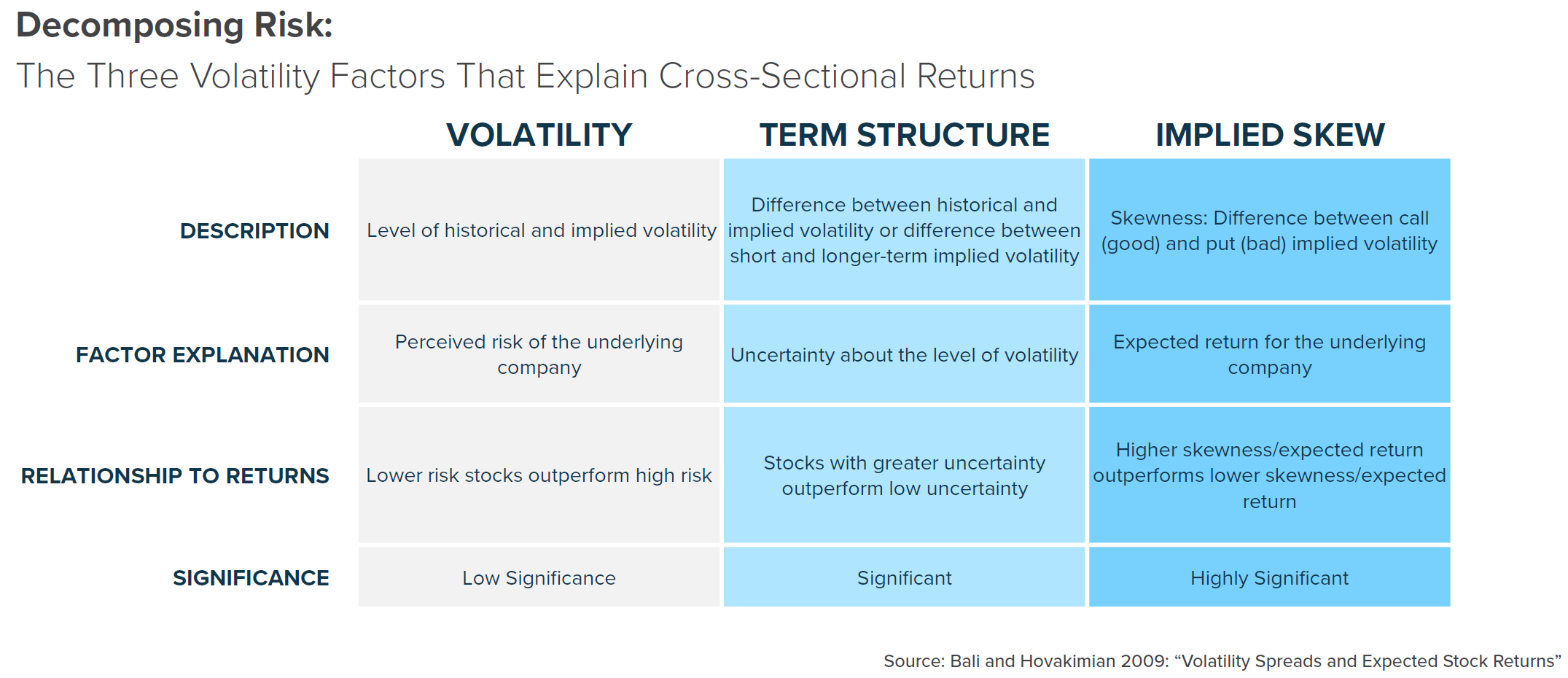There are many different ways to estimate returns using quantitative data. The conventional approach is to use a ‘factor model’ which uses predictor variables weighted in some optimal combination to estimate absolute or relative returns. Most factor models combine company fundamental or technical (price and volume) data. Traditional smart beta factors used within these models by both industry practitioners and academia are momentum, value, size, volatility/beta and quality. A good example is the ‘Fama-French’ 3 and 5-factor models that have become the gold standard for explaining the cross-section of returns. The same models are also commonly used for benchmarking purposes to determine if a new proposed factor has a truly unique source of alpha.  Apart from the traditional factors, there is a substantial and growing body of research which shows that the ‘volatility surface’ is also a rich source of information for generating return estimates. Given that historical volatility and beta are already well-established factors, the more information-rich and ‘forward looking’ volatility surface is a logical place to examine for newer and better factors.

What is the Volatility Surface?

A good analogy for the volatility surface is that it is a 3D map of the market’s estimated odds for where a stock or asset price is likely to be at some point in the future.  The volatility surface is derived using equity or asset option data where the price of options can be used to ‘imply’ or reverse-engineer the expectations for risk/reward or volatility using mathematical option-pricing models.  If we look at the spectrum of option market data for each company, typically you will see this ‘implied volatility’ for call options (bets that a stock will go up)  and put options (bets that a stock will go down) with different strike prices (moneyness or the price at which the option can make a profit) and different expirations (or time to maturity). Collectively, these data points are called ‘The Volatility Surface’ – capturing the expected probability distribution for the stock across time.Academic research shows that we can estimate returns using this data by comparing various metrics calculated from the volatility surface and creating a factor model.

There are various advantages to using option-implied volatility versus traditional ‘realized’ measures. Historical Volatility is calculated using historical daily returns and by definition, is a backward-looking estimate. Implied Volatility is determined using equity option market data and is the forward- looking expectation of volatility in the future. No one drives by looking through the rear-view mirror for what has happened so why would we use historical volatility in the first place? It is only logical that forward-looking data may be more useful. One of the main reasons for this is that implied volatility is a market-based estimate and therefore incorporates all available information to traders and investors at a particular point in time.

Research by Krause and Behrend (‘Implied Volatility Factors’) shows that implied volatility (90-day) incorporates a wide range of information not captured by historical data such as the following fundamental and technical factors:

• Size
• Volume/Turnover
• Dividend Yields
• Beta
• Valuations
• Quality
• Estimated Growth Rates
• Momentum
• Leverage

Another advantage of using implied volatility is it is a ‘parameter-free’ forecast model.  In other words, the most important, or relevant factors, are already embedded and therefore don’t require calibration. In contrast, traditional historical volatility models or even volatility ‘forecast’ models, which ironically use historical data, require that a person needs to choose a set of parameters which introduces more bias into estimation. The same can be said for all factor or multi-factor models. The caveat is that you still need to choose a parameter such as the days to expiration for implied volatility.  The clear advantage is that the option data compresses and self-calibrates the weighting of a lot of different pieces of information.

How to Use the Volatility Surface to Estimate Returns

There are a lot of different ways to use the volatility surface data: you have implied volatility for both call and put options, as well as different degrees of ‘moneyness’ (delta or the probability that the option will make a profit) and different maturities or months/years to expiration. In other words, there exist several different dimensions to analyze the data. The most common and accepted methodology for reducing dimensionality is principal component analysis or PCA for short. This advanced quantitative method quickly distills a range of possibilities to use information into the most important and unique factors. We can analyze the significance of these individual factors in terms of their ability to explain or predict stock returns. Finally, we can simplify these factors into more basic indicators by matching their correlation to the original and more complex factors. These new simplified factors can be used for stock ranking or in multi-factor models.

Bali and Hovakimian (Volatility Spreads and Expected Stock Returns, 2009) were the first to take this basic approach to decomposing the volatility surface. Their paper investigated whether realized (historical) and implied volatilities (option-derived) of individual stocks can predict the cross-sectional variation in expected returns.

They identified three distinct factors that captured the volatility surface using their PCA analysis:

1. Volatility: The level of volatility using either historical or implied volatility (option-derived).
2. Volatility Uncertainty or Term-Structure: this is the difference between historical and implied volatility.
3. Volatility Spread or Implied Skew: the difference between ‘good’/ ‘upside’ (call option implied vol) and ‘bad’ / ‘downside’ (put option implied vol) volatility.What they found was that the level of volatility, whether implied or historical, had no significant value in forecasting stock returns when controlling for the other two factors. They found that the difference between historical and implied volatility – what I will call a ‘term-structure’ factor- has a negative relationship to expected returns.  When future estimated volatility is much higher than historical volatility this suggests a high degree of uncertainty about future performance. Stocks with high uncertainty tend to outperform stocks with low uncertainty.